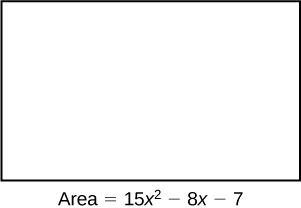# 1.6 Rational expressions  (Page 4/6)

 Page 4 / 6

For the following exercises, divide the rational expressions.

$\frac{3{y}^{2}-7y-6}{2{y}^{2}-3y-9}÷\frac{{y}^{2}+y-2}{2{y}^{2}+y-3}$

$\frac{6{p}^{2}+p-12}{8{p}^{2}+18p+9}÷\frac{6{p}^{2}-11p+4}{2{p}^{2}+11p-6}$

$\frac{p+6}{4p+3}$

$\frac{{q}^{2}-9}{{q}^{2}+6q+9}÷\frac{{q}^{2}-2q-3}{{q}^{2}+2q-3}$

$\frac{18{d}^{2}+77d-18}{27{d}^{2}-15d+2}÷\frac{3{d}^{2}+29d-44}{9{d}^{2}-15d+4}$

$\frac{2d+9}{d+11}$

$\frac{16{x}^{2}+18x-55}{32{x}^{2}-36x-11}÷\frac{2{x}^{2}+17x+30}{4{x}^{2}+25x+6}$

$\frac{144{b}^{2}-25}{72{b}^{2}-6b-10}÷\frac{18{b}^{2}-21b+5}{36{b}^{2}-18b-10}$

$\frac{12b+5}{3b-1}$

$\frac{16{a}^{2}-24a+9}{4{a}^{2}+17a-15}÷\frac{16{a}^{2}-9}{4{a}^{2}+11a+6}$

$\frac{22{y}^{2}+59y+10}{12{y}^{2}+28y-5}÷\frac{11{y}^{2}+46y+8}{24{y}^{2}-10y+1}$

$\frac{4y-1}{y+4}$

$\frac{9{x}^{2}+3x-20}{3{x}^{2}-7x+4}÷\frac{6{x}^{2}+4x-10}{{x}^{2}-2x+1}$

For the following exercises, add and subtract the rational expressions, and then simplify.

$\frac{4}{x}+\frac{10}{y}$

$\frac{10x+4y}{xy}$

$\frac{12}{2q}-\frac{6}{3p}$

$\frac{4}{a+1}+\frac{5}{a-3}$

$\frac{9a-7}{{a}^{2}-2a-3}$

$\frac{c+2}{3}-\frac{c-4}{4}$

$\frac{y+3}{y-2}+\frac{y-3}{y+1}$

$\frac{2{y}^{2}-y+9}{{y}^{2}-y-2}$

$\frac{x-1}{x+1}-\frac{2x+3}{2x+1}$

$\frac{3z}{z+1}+\frac{2z+5}{z-2}$

$\frac{5{z}^{2}+z+5}{{z}^{2}-z-2}$

$\frac{4p}{p+1}-\frac{p+1}{4p}$

$\frac{x}{x+1}+\frac{y}{y+1}$

$\frac{x+2xy+y}{x+xy+y+1}$

For the following exercises, simplify the rational expression.

$\frac{\frac{6}{y}-\frac{4}{x}}{y}$

$\frac{\frac{2}{a}+\frac{7}{b}}{b}$

$\frac{2b+7a}{a{b}^{2}}$

$\frac{\frac{x}{4}-\frac{p}{8}}{p}$

$\frac{\frac{3}{a}+\frac{b}{6}}{\frac{2b}{3a}}$

$\frac{18+ab}{4b}$

$\frac{\frac{3}{x+1}+\frac{2}{x-1}}{\frac{x-1}{x+1}}$

$\frac{\frac{a}{b}-\frac{b}{a}}{\frac{a+b}{ab}}$

$a-b$

$\frac{\frac{2x}{3}+\frac{4x}{7}}{\frac{x}{2}}$

$\frac{\frac{2c}{c+2}+\frac{c-1}{c+1}}{\frac{2c+1}{c+1}}$

$\frac{3{c}^{2}+3c-2}{2{c}^{2}+5c+2}$

$\frac{\frac{x}{y}-\frac{y}{x}}{\frac{x}{y}+\frac{y}{x}}$

## Real-world applications

Brenda is placing tile on her bathroom floor. The area of the floor is $\text{\hspace{0.17em}}15{x}^{2}-8x-7\text{\hspace{0.17em}}$ ft 2 . The area of one tile is $\text{\hspace{0.17em}}{x}^{2}-2x+1{\text{ft}}^{2}.\text{\hspace{0.17em}}$ To find the number of tiles needed, simplify the rational expression: $\text{\hspace{0.17em}}\frac{15{x}^{2}-8x-7}{{x}^{2}-2x+1}.$$\frac{15x+7}{x-1}$

The area of Sandy’s yard is $\text{\hspace{0.17em}}25{x}^{2}-625\text{\hspace{0.17em}}$ ft 2 . A patch of sod has an area of $\text{\hspace{0.17em}}{x}^{2}-10x+25\text{\hspace{0.17em}}$ ft 2 . Divide the two areas and simplify to find how many pieces of sod Sandy needs to cover her yard.

Aaron wants to mulch his garden. His garden is $\text{\hspace{0.17em}}{x}^{2}+18x+81\text{\hspace{0.17em}}$ ft 2 . One bag of mulch covers $\text{\hspace{0.17em}}{x}^{2}-81\text{\hspace{0.17em}}$ ft 2 . Divide the expressions and simplify to find how many bags of mulch Aaron needs to mulch his garden.

$\frac{x+9}{x-9}$

## Extensions

For the following exercises, perform the given operations and simplify.

$\frac{{x}^{2}+x-6}{{x}^{2}-2x-3}\cdot \frac{2{x}^{2}-3x-9}{{x}^{2}-x-2}÷\frac{10{x}^{2}+27x+18}{{x}^{2}+2x+1}$

$\frac{\frac{3{y}^{2}-10y+3}{3{y}^{2}+5y-2}\cdot \frac{2{y}^{2}-3y-20}{2{y}^{2}-y-15}}{y-4}$

$\frac{1}{y+2}$

$\frac{\frac{4a+1}{2a-3}+\frac{2a-3}{2a+3}}{\frac{4{a}^{2}+9}{a}}$

$\frac{{x}^{2}+7x+12}{{x}^{2}+x-6}÷\frac{3{x}^{2}+19x+28}{8{x}^{2}-4x-24}÷\frac{2{x}^{2}+x-3}{3{x}^{2}+4x-7}$

$4$

## Real Numbers: Algebra Essentials

For the following exercises, perform the given operations.

${\left(5-3\cdot 2\right)}^{2}-6$

$-5$

$64÷\left(2\cdot 8\right)+14÷7$

$2\cdot {5}^{2}+6÷2$

53

For the following exercises, solve the equation.

$5x+9=-11$

$2y+{4}^{2}=64$

$y=24$

For the following exercises, simplify the expression.

$9\left(y+2\right)÷3\cdot 2+1$

$3m\left(4+7\right)-m$

$32m$

For the following exercises, identify the number as rational, irrational, whole, or natural. Choose the most descriptive answer.

11

0

whole

$\frac{5}{6}$

$\sqrt{11}$

irrational

## Exponents and Scientific Notation

For the following exercises, simplify the expression.

${2}^{2}\cdot {2}^{4}$

$\frac{{4}^{5}}{{4}^{3}}$

$16$

${\left(\frac{{a}^{2}}{{b}^{3}}\right)}^{4}$

$\frac{6{a}^{2}\cdot {a}^{0}}{2{a}^{-4}}$

${a}^{6}$

$\frac{{\left(xy\right)}^{4}}{{y}^{3}}\cdot \frac{2}{{x}^{5}}$

$\frac{{4}^{-2}{x}^{3}{y}^{-3}}{2{x}^{0}}$

$\frac{{x}^{3}}{32{y}^{3}}$

${\left(\frac{2{x}^{2}}{y}\right)}^{-2}$

$\left(\frac{16{a}^{3}}{{b}^{2}}\right){\left(4a{b}^{-1}\right)}^{-2}$

$a$

Write the number in standard notation: $\text{\hspace{0.17em}}2.1314\text{\hspace{0.17em}}×\text{\hspace{0.17em}}{10}^{-6}$

Write the number in scientific notation: 16,340,000

$1.634\text{\hspace{0.17em}}×\text{\hspace{0.17em}}{10}^{7}$

For the following exercises, find the principal square root.

$\sqrt{121}$

$\sqrt{196}$

14

$\sqrt{361}$

$\sqrt{75}$

$5\sqrt{3}$

$\sqrt{162}$

$\sqrt{\frac{32}{25}}$

$\frac{4\sqrt{2}}{5}$

$\sqrt{\frac{80}{81}}$

$\sqrt{\frac{49}{1250}}$

$\frac{7\sqrt{2}}{50}$

$\frac{2}{4+\sqrt{2}}$

$4\sqrt{3}+6\sqrt{3}$

$10\sqrt{3}$

$12\sqrt{5}-13\sqrt{5}$

$\sqrt{-243}$

$-3$

$\frac{\sqrt{250}}{\sqrt{-8}}$

## Polynomials

For the following exercises, perform the given operations and simplify.

$\left(3{x}^{3}+2x-1\right)+\left(4{x}^{2}-2x+7\right)$

$3{x}^{3}+4{x}^{2}+6$

$\left(2y+1\right)-\left(2{y}^{2}-2y-5\right)$

$\left(2{x}^{2}+3x-6\right)+\left(3{x}^{2}-4x+9\right)$

$5{x}^{2}-x+3$

$\left(6{a}^{2}+3a+10\right)-\left(6{a}^{2}-3a+5\right)$

$\left(k+3\right)\left(k-6\right)$

${k}^{2}-3k-18$

$\left(2h+1\right)\left(3h-2\right)$

$\left(x+1\right)\left({x}^{2}+1\right)$

${x}^{3}+{x}^{2}+x+1$

$\left(m-2\right)\left({m}^{2}+2m-3\right)$

$\left(a+2b\right)\left(3a-b\right)$

$3{a}^{2}+5ab-2{b}^{2}$

$\left(x+y\right)\left(x-y\right)$

## Factoring Polynomials

For the following exercises, find the greatest common factor.

$81p+9pq-27{p}^{2}{q}^{2}$

$9p$

$12{x}^{2}y+4x{y}^{2}-18xy$

$88{a}^{3}b+4{a}^{2}b-144{a}^{2}$

$4{a}^{2}$

For the following exercises, factor the polynomial.

$2{x}^{2}-9x-18$

$8{a}^{2}+30a-27$

$\left(4a-3\right)\left(2a+9\right)$

${d}^{2}-5d-66$

${x}^{2}+10x+25$

${\left(x+5\right)}^{2}$

${y}^{2}-6y+9$

$4{h}^{2}-12hk+9{k}^{2}$

${\left(2h-3k\right)}^{2}$

$361{x}^{2}-121$

${p}^{3}+216$

$\left(p+6\right)\left({p}^{2}-6p+36\right)$

$8{x}^{3}-125$

$64{q}^{3}-27{p}^{3}$

$\left(4q-3p\right)\left(16{q}^{2}+12pq+9{p}^{2}\right)$

$4x{\left(x-1\right)}^{-\frac{1}{4}}+3{\left(x-1\right)}^{\frac{3}{4}}$

$3p{\left(p+3\right)}^{\frac{1}{3}}-8{\left(p+3\right)}^{\frac{4}{3}}$

${\left(p+3\right)}^{\frac{1}{3}}\left(-5p-24\right)$

$4r{\left(2r-1\right)}^{-\frac{2}{3}}-5{\left(2r-1\right)}^{\frac{1}{3}}$

## Rational Expressions

For the following exercises, simplify the expression.

$\frac{{x}^{2}-x-12}{{x}^{2}-8x+16}$

$\frac{x+3}{x-4}$

$\frac{4{y}^{2}-25}{4{y}^{2}-20y+25}$

$\frac{2{a}^{2}-a-3}{2{a}^{2}-6a-8}\cdot \frac{5{a}^{2}-19a-4}{10{a}^{2}-13a-3}$

$\frac{1}{2}$

$\frac{d-4}{{d}^{2}-9}\cdot \frac{d-3}{{d}^{2}-16}$

$\frac{{m}^{2}+5m+6}{2{m}^{2}-5m-3}÷\frac{2{m}^{2}+3m-9}{4{m}^{2}-4m-3}$

$\frac{m+2}{m-3}$

$\frac{4{d}^{2}-7d-2}{6{d}^{2}-17d+10}÷\frac{8{d}^{2}+6d+1}{6{d}^{2}+7d-10}$

$\frac{10}{x}+\frac{6}{y}$

$\frac{6x+10y}{xy}$

$\frac{12}{{a}^{2}+2a+1}-\frac{3}{{a}^{2}-1}$

$\frac{\frac{1}{d}+\frac{2}{c}}{\frac{6c+12d}{dc}}$

$\frac{1}{6}$

$\frac{\frac{3}{x}-\frac{7}{y}}{\frac{2}{x}}$

## Chapter practice test

For the following exercises, identify the number as rational, irrational, whole, or natural. Choose the most descriptive answer.

$-13$

rational

$\sqrt{2}$

For the following exercises, evaluate the equations.

$2\left(x+3\right)-12=18$

$x=12$

$y{\left(3+3\right)}^{2}-26=10$

Write the number in standard notation: $3.1415\text{\hspace{0.17em}}×\text{\hspace{0.17em}}{10}^{6}$

3,141,500

Write the number in scientific notation: 0.0000000212.

For the following exercises, simplify the expression.

$-2\cdot {\left(2+3\cdot 2\right)}^{2}+144$

$16$

$4\left(x+3\right)-\left(6x+2\right)$

${3}^{5}\cdot {3}^{-3}$

9

${\left(\frac{2}{3}\right)}^{3}$

$\frac{8{x}^{3}}{{\left(2x\right)}^{2}}$

$2x$

$\left(16{y}^{0}\right)2{y}^{-2}$

$\sqrt{441}$

21

$\sqrt{490}$

$\sqrt{\frac{9x}{16}}$

$\frac{3\sqrt{x}}{4}$

$\frac{\sqrt{121{b}^{2}}}{1+\sqrt{b}}$

$6\sqrt{24}+7\sqrt{54}-12\sqrt{6}$

$21\sqrt{6}$

$\frac{\sqrt{-8}}{\sqrt{625}}$

$\left(13{q}^{3}+2{q}^{2}-3\right)-\left(6{q}^{2}+5q-3\right)$

$13{q}^{3}-4{q}^{2}-5q$

$\left(6{p}^{2}+2p+1\right)+\left(9{p}^{2}-1\right)$

$\left(n-2\right)\left({n}^{2}-4n+4\right)$

${n}^{3}-6{n}^{2}+12n-8$

$\left(a-2b\right)\left(2a+b\right)$

For the following exercises, factor the polynomial.

$16{x}^{2}-81$

$\left(4x+9\right)\left(4x-9\right)$

${y}^{2}+12y+36$

$27{c}^{3}-1331$

$\left(3c-11\right)\left(9{c}^{2}+33c+121\right)$

$3x{\left(x-6\right)}^{-\frac{1}{4}}+2{\left(x-6\right)}^{\frac{3}{4}}$

For the following exercises, simplify the expression.

$\frac{2{z}^{2}+7z+3}{{z}^{2}-9}\cdot \frac{4{z}^{2}-15z+9}{4{z}^{2}-1}$

$\frac{4z-3}{2z-1}$

$\frac{x}{y}+\frac{2}{x}$

$\frac{\frac{a}{2b}-\frac{2b}{9a}}{\frac{3a-2b}{6a}}$

$\frac{3a+2b}{3b}$

A laser rangefinder is locked on a comet approaching Earth. The distance g(x), in kilometers, of the comet after x days, for x in the interval 0 to 30 days, is given by g(x)=250,000csc(π30x). Graph g(x) on the interval [0, 35]. Evaluate g(5)  and interpret the information. What is the minimum distance between the comet and Earth? When does this occur? To which constant in the equation does this correspond? Find and discuss the meaning of any vertical asymptotes.
The sequence is {1,-1,1-1.....} has
how can we solve this problem
Sin(A+B) = sinBcosA+cosBsinA
Prove it
Eseka
Eseka
hi
Joel
June needs 45 gallons of punch. 2 different coolers. Bigger cooler is 5 times as large as smaller cooler. How many gallons in each cooler?
7.5 and 37.5
Nando
find the sum of 28th term of the AP 3+10+17+---------
I think you should say "28 terms" instead of "28th term"
Vedant
the 28th term is 175
Nando
192
Kenneth
if sequence sn is a such that sn>0 for all n and lim sn=0than prove that lim (s1 s2............ sn) ke hole power n =n
write down the polynomial function with root 1/3,2,-3 with solution
if A and B are subspaces of V prove that (A+B)/B=A/(A-B)
write down the value of each of the following in surd form a)cos(-65°) b)sin(-180°)c)tan(225°)d)tan(135°)
Prove that (sinA/1-cosA - 1-cosA/sinA) (cosA/1-sinA - 1-sinA/cosA) = 4
what is the answer to dividing negative index
In a triangle ABC prove that. (b+c)cosA+(c+a)cosB+(a+b)cisC=a+b+c.
give me the waec 2019 questionsByByBy Anonymous User